Select Page

Miles to kilometers, celsius to Fahrenheit, and Google Docs to word are just some of the conversions we all do in our day-to-day life. When we fly overseas (hello pre-COVID days) or work with a company with different sharing methods, we often find ourselves converting in the ‘opposite’ direction. It may be a slower process due to lack of practice but there’s no doubt it is possible. If only that was the case of DMatrix and NumPy formats.

For those scratching their heads wondering what DMatrix is, it’s an internal data format used by the XGBoost library (open-source gradient boosting tree framework for C ++, Java, Python,etc.) The Dmatrix format is optimized for both memory efficiency and training speed.

### One way ticket to DMatrix?

The nice and easy flow XGBoost provides out of the box is converting common data structures like NumPy array, scipy.sparse and pd.DataFrame to Dmatrix. But unlike the conversions mentioned above, this one is considered to be a one-way-ticket.
But what should a user do if they need to fly back from DMatrix to NumPy array? That’s exactly what we wanted to figure out while developing our solution for ML model productization.

TL;DR – pip install dmatrix2np (go to the project)

Below you will find a step by step of our research process. We recommend reading it before integrating it into your live model.

### Why going NumPy?

Before we explain how we cracked it, let’s say why should you bother. Unlike DMatrix which doesn’t provide access to features data, NumPy provides direct python interfaces for accessing and manipulating the data. NumPy is a long-standing industry standard that is well known for its efficiency and power.

When we set to convert data from DMatrix to NumPy format we googled out that the common belief is “DMatrix is a one-way street: once you get DMatrix, you cannot get back its content as NumPy”. We love challenges and as explained we had a good reason to make this effort so we started exploring how.

### Step 1: Diving in, RTFM

Since XGBoost is an open-source we started by reading the implementation of DMatrix in the XGBoost Python package. We first looked at the constructor – according to the documentation, it is able to get a variety of data types among them NumPy and convert them to the DMatrix data type. We thought that if we understood the conversion logic in one direction we might also find a simple and quick way to return.

 1 2 3 4 5 6 7 8 9 10 11 12 13 14 15 16 17 18 19 20 21 22 23 24 25 26 27 28 29 30 31 32 33 34 35 36 37 38 39 40 41 42 43 44 45 46 47 48 49 50 51 52 53 54 55 56 57 58 59 60 61 62 63 64 `class` `DMatrix(``object``):` ` `  `    ``_feature_names ``=` `None`  `# for previous version's pickle` `    ``_feature_types ``=` `None` ` `  `    ``def` `__init__(``self``, data, label``=``None``, weight``=``None``, base_margin``=``None``,` `                 ``missing``=``None``,` `                 ``silent``=``False``,` `                 ``feature_names``=``None``,` `                 ``feature_types``=``None``,` `                 ``nthread``=``None``):` `        ``# force into void_p, mac need to pass things in as void_p` `        ``if` `data ``is` `None``:` `            ``self``.handle ``=` `None` ` `  `            ``if` `feature_names ``is` `not` `None``:` `                ``self``._feature_names ``=` `feature_names` `            ``if` `feature_types ``is` `not` `None``:` `                ``self``._feature_types ``=` `feature_types` `            ``return` ` `  `        ``if` `isinstance``(data, ``list``):` `            ``raise` `TypeError(``'Input data can not be a list.'``)` ` `  `        ``data, feature_names, feature_types ``=` `_convert_dataframes(` `            ``data, feature_names, feature_types` `        ``)` `        ``missing ``=` `missing ``if` `missing ``is` `not` `None` `else` `np.nan` `        ``nthread ``=` `nthread ``if` `nthread ``is` `not` `None` `else` `1` ` `  `        ``if` `isinstance``(data, (STRING_TYPES, os_PathLike)):` `            ``handle ``=` `ctypes.c_void_p()` `            ``_check_call(_LIB.XGDMatrixCreateFromFile(c_str(os_fspath(data)),` `                                                     ``ctypes.c_int(silent),` `                                                     ``ctypes.byref(handle)))` `            ``self``.handle ``=` `handle` `        ``elif` `isinstance``(data, scipy.sparse.csr_matrix):` `            ``self``._init_from_csr(data)` `        ``elif` `isinstance``(data, scipy.sparse.csc_matrix):` `            ``self``._init_from_csc(data)` `        ``elif` `isinstance``(data, np.ndarray):` `            ``self``._init_from_npy2d(data, missing, nthread)` `        ``elif` `lazy_isinstance(data, ``'datatable'``, ``'Frame'``):` `            ``self``._init_from_dt(data, nthread)` `        ``elif` `hasattr``(data, ``"__cuda_array_interface__"``):` `            ``self``._init_from_array_interface(data, missing, nthread)` `        ``elif` `CUDF_INSTALLED ``and` `isinstance``(data, CUDF_DataFrame):` `            ``self``._init_from_array_interface_columns(data, missing, nthread)` `        ``else``:` `            ``try``:` `                ``csr ``=` `scipy.sparse.csr_matrix(data)` `                ``self``._init_from_csr(csr)` `            ``except` `Exception:` `                ``raise` `TypeError(``'can not initialize DMatrix from'` `                                ``' {}'``.``format``(``type``(data).__name__)) ` `        ``if` `label ``is` `not` `None``:` `            ``self``.set_label(label)` `        ``if` `weight ``is` `not` `None``:` `            ``self``.set_weight(weight)` `        ``if` `base_margin ``is` `not` `None``:` `            ``self``.set_base_margin(base_margin)` ` `  `        ``self``.feature_names ``=` `feature_names` `        ``self``.feature_types ``=` `feature_types`

We saw that for each data structure, the constructor uses a dedicated internal conversion method. Let’s take a look at the ‘init from npy2d’ for example:

 1 2 3 4 5 6 7 8 9 10 11 12 13 14 `def` `_init_from_npy2d(``self``, mat, missing, nthread):` `    ``if` `len``(mat.shape) !``=` `2``:` `        ``raise` `ValueError(``'Expecting 2 dimensional numpy.ndarray, got: '``,` `                            ``mat.shape)` `    ``data ``=` `np.array(mat.reshape(mat.size), copy``=``False``, dtype``=``np.float32)` `    ``handle ``=` `ctypes.c_void_p()` `    ``_check_call(_LIB.XGDMatrixCreateFromMat_omp(` `        ``data.ctypes.data_as(ctypes.POINTER(ctypes.c_float)),` `        ``c_bst_ulong(mat.shape[``0``]),` `        ``c_bst_ulong(mat.shape[``1``]),` `        ``ctypes.c_float(missing),` `        ``ctypes.byref(handle),` `        ``ctypes.c_int(nthread)))` `    ``self``.handle ``=` `handle`

The method expects a 2-dimensional NumPy array. It flattens the array by rows, ensuring that the data type is float32 and then call an API implemented in C++ using python ctypes. Finally – it sets the DMatrix to handle attribute with the value returned from the low-level API.
This high-level logic is true for all the other conversion methods.

We then searched for a method or an attribute that stores the features data or calls a low-level API that is capable of receiving a handle and providing access to the data.

As it happens too often in life we couldn’t find the exact thing we were looking for but we found something else. The labels data can be obtained in a simple way and there’s a method that can export the DMatrix object into a binary file format:

 1 2 3 4 `def` `save_binary(``self``, fname, silent``=``True``):` `    ``_check_call(_LIB.XGDMatrixSaveBinary(``self``.handle,` `                                         ``c_str(os_fspath(fname)),` `                                         ``ctypes.c_int(silent)))`

### Step 2: Low-level logic

Once we realized that the actual conversion and data access functionality is most likely implemented in the C++ API, we went to read the logic implemented in C++.
We were hoping to find an API that enables conversion to NumPy (HA!), or at least access to some DMatrix features data.

The API methods are implemented in the c_api.cc file. Unfortunately, we’ve found only methods that allow access to the meta info. For example, this method with the long and promising name ‘XGDMatrixGetFloatInfo’ which allows access only to specific data from the Meta info:

 1 2 3 4 5 6 7 8 9 10 11 12 13 14 15 16 17 18 19 20 21 22 23 24 25 `XGB_DLL ``int` `XGDMatrixGetFloatInfo(``const` `DMatrixHandle handle,` `                                  ``const` `char``* field,` `                                  ``xgboost::bst_ulong* out_len,` `                                  ``const` `bst_float** out_dptr) {` `  ``API_BEGIN();` `  ``CHECK_HANDLE();` `  ``const` `MetaInfo&amp; info = ``static_cast``&lt;std::shared_ptr&lt;DMatrix&gt;*&gt;(handle)-&gt;get()-&gt;Info();` `  ``const` `std::vector&lt;bst_float&gt;* vec = ``nullptr``;` `  ``if` `(!std::``strcmp``(field, ``"label"``)) {` `    ``vec = &amp;info.labels_.HostVector();` `  ``} ``else` `if` `(!std::``strcmp``(field, ``"weight"``)) {` `    ``vec = &amp;info.weights_.HostVector();` `  ``} ``else` `if` `(!std::``strcmp``(field, ``"base_margin"``)) {` `    ``vec = &amp;info.base_margin_.HostVector();` `  ``} ``else` `if` `(!std::``strcmp``(field, ``"label_lower_bound"``)) {` `    ``vec = &amp;info.labels_lower_bound_.HostVector();` `  ``} ``else` `if` `(!std::``strcmp``(field, ``"label_upper_bound"``)) {` `    ``vec = &amp;info.labels_upper_bound_.HostVector();` `  ``} ``else` `{` `    ``LOG(FATAL) &lt;&lt; ``"Unknown float field name "` `&lt;&lt; field;` `  ``}` `  ``*out_len = ``static_cast``&lt;xgboost::bst_ulong&gt;(vec-&gt;size());  ``// NOLINT` `  ``*out_dptr = dmlc::BeginPtr(*vec);` `  ``API_END();` `}`

So, we tried to understand the low-level conversion logic implemented in XGBoost C++ code, aiming to figure an algorithm that will perform the reverse conversion and to implement it.

At first glance, the logic seemed clear, but we already knew that direct reading from the handle using high-level methods is not the right way to go about this reverse-engineering. We suspected that a library that reads directly from the handle would suffer from two major drawbacks — developing part of the library in C++ would mean increasing the development and distribution time. Worst, we’d have to examine XGBoost code for each new distributed version and release a fast patch for any small change in the data structure stored in the handle.

But wait a minute, what about the option of exporting DMatrix to a binary file?

By its nature, an exported binary file format remains more consistent (precisely because developers would go far not to break support between versions), and parsing the structure could also be done safely in Python.

### Step 3: Could the binary format data be the answer?

The obvious starting point was the Python function that provides the conversion to the binary format – ‘save_binary’. Like the previous functions we’ve examined, there was just a reference to the C ++ API directly.

 1 2 3 4 `def` `save_binary(``self``, fname, silent``=``True``):` `    ``_check_call(_LIB.XGDMatrixSaveBinary(``self``.handle,` `                                          ``c_str(os_fspath(fname)),` `                                          ``ctypes.c_int(silent)))`

We moved on to the C ++ API, which directly referred us to a method called ‘SaveToLocalFile’ of the SimpleDMatrix class.

 1 2 3 4 5 6 7 8 9 10 11 12 `XGB_DLL ``int` `XGDMatrixSaveBinary(DMatrixHandle handle, ``const` `char``* fname,` `                                ``int` `silent) {` `  ``API_BEGIN();` `  ``CHECK_HANDLE();` `  ``auto` `dmat = ``static_cast``&lt;std::shared_ptr&lt;DMatrix&gt;*&gt;(handle)-&gt;get();` `  ``if` `(data::SimpleDMatrix* derived = ``dynamic_cast``&lt;data::SimpleDMatrix*&gt;(dmat)) {` `    ``derived-&gt;SaveToLocalFile(fname);` `  ``} ``else` `{` `    ``LOG(FATAL) &lt;&lt; ``"binary saving only supported by SimpleDMatrix"``;` `  ``}` `  ``API_END();` `}`
 1 2 3 4 5 6 7 8 `void` `SimpleDMatrix::SaveToLocalFile(``const` `std::string&amp; fname) {` `    ``std::unique_ptr&lt;dmlc::Stream&gt; fo(dmlc::Stream::Create(fname.c_str(), ``"w"``));` `    ``int` `tmagic = kMagic;` `    ``fo-&gt;Write(&amp;tmagic, ``sizeof``(tmagic));` `    ``info_.SaveBinary(fo.get());` `    ``fo-&gt;Write(sparse_page_.offset.HostVector());` `    ``fo-&gt;Write(sparse_page_.data.HostVector());` `}`

From this method we learned that the binary structure can be divided into 4 parts:

1. Magic
2. Meta Info
3. Offset Vector
4. Data Vector

So let’s start with the magic – simple integer constant set to static const int kMagic = 0xffffab02;
Thi value is consistent between all XGBoost python package versions we checked (0.80 to 1.0.2)

The meta info is a bit more complicated:

 1 2 3 4 5 6 7 8 9 10 11 12 13 14 15 16 17 18 19 20 21 22 23 `void` `MetaInfo::SaveBinary(dmlc::Stream *fo) ``const` `{` `  ``Version::Save(fo);` `  ``fo-&gt;Write(kNumField);` `  ``int` `field_cnt = 0;  ``// make sure we are actually writing kNumField fields` ` `  `  ``SaveScalarField(fo, ``u8"num_row"``, DataType::kUInt64, num_row_); ++field_cnt;` `  ``SaveScalarField(fo, ``u8"num_col"``, DataType::kUInt64, num_col_); ++field_cnt;` `  ``SaveScalarField(fo, ``u8"num_nonzero"``, DataType::kUInt64, num_nonzero_); ++field_cnt;` `  ``SaveVectorField(fo, ``u8"labels"``, DataType::kFloat32,` `                  ``{labels_.Size(), 1}, labels_); ++field_cnt;` `  ``SaveVectorField(fo, ``u8"group_ptr"``, DataType::kUInt32,` `                  ``{group_ptr_.size(), 1}, group_ptr_); ++field_cnt;` `  ``SaveVectorField(fo, ``u8"weights"``, DataType::kFloat32,` `                  ``{weights_.Size(), 1}, weights_); ++field_cnt;` `  ``SaveVectorField(fo, ``u8"base_margin"``, DataType::kFloat32,` `                  ``{base_margin_.Size(), 1}, base_margin_); ++field_cnt;` `  ``SaveVectorField(fo, ``u8"labels_lower_bound"``, DataType::kFloat32,` `                  ``{labels_lower_bound_.Size(), 1}, labels_lower_bound_); ++field_cnt;` `  ``SaveVectorField(fo, ``u8"labels_upper_bound"``, DataType::kFloat32,` `                  ``{labels_upper_bound_.Size(), 1}, labels_upper_bound_); ++field_cnt;` ` `  `  ``CHECK_EQ(field_cnt, kNumField) &lt;&lt; ``"Wrong number of fields"``;` `}`

It is made of ‘version’ structure – a ‘version:’ string + package version for the newer XGBoost versions (1.0.0 +) or a simple integer in the earlier versions.

Immediately after the version structure/field comes the meta info fields.
First, there’s an indicator for the number of fields (only in the versions 1.0.0+) and then there are information fields of two types – scalar which is a one-dimensional value in a given type or vector which is a data list of items from a specific type.

In the newer versions, each field is represented by a string of the field name, then the field type, whether the field is scalar, sizing for vector fields, and then the raw information.
In older versions, the information is simply chained one by one.

 1 2 3 4 5 6 7 8 9 10 11 12 13 14 15 16 17 18 19 20 `template` `&lt;``typename` `T&gt;` `void` `SaveScalarField(dmlc::Stream *strm, ``const` `std::string &amp;name,` `                     ``xgboost::DataType type, ``const` `T &amp;field) {` `  ``strm-&gt;Write(name);` `  ``strm-&gt;Write(``static_cast``&lt;``uint8_t``&gt;(type));` `  ``strm-&gt;Write(``true``);  ``// is_scalar=True` `  ``strm-&gt;Write(field);` `}` ` `  `template` `&lt;``typename` `T&gt;` `void` `SaveVectorField(dmlc::Stream *strm, ``const` `std::string &amp;name,` `                     ``xgboost::DataType type, std::pair&lt;``uint64_t``, ``uint64_t``&gt; shape,` `                     ``const` `std::vector&lt;T&gt;&amp; field) {` `  ``strm-&gt;Write(name);` `  ``strm-&gt;Write(``static_cast``&lt;``uint8_t``&gt;(type));` `  ``strm-&gt;Write(``false``);  ``// is_scalar=False` `  ``strm-&gt;Write(shape.first);` `  ``strm-&gt;Write(shape.second);` `  ``strm-&gt;Write(field);` `}`

The write function is also interesting – in the event of writing a string to the binary file, before the expected string there is a size indicator (pascal string), and in the event of vector appearance, there is a prefix to indicate the number of items in the vector.

 1 2 3 4 5 6 `struct` `ComposeVectorHandler {` `  ``inline` `static` `void` `Write(Stream *strm, ``const` `std::vector&lt;T&gt; &amp;vec) {` `    ``uint64_t` `sz = ``static_cast``&lt;``uint64_t``&gt;(vec.size());` `    ``strm-&gt;Write&lt;``uint64_t``&gt;(sz);` `    ``strm-&gt;WriteArray(dmlc::BeginPtr(vec), vec.size());` `  ``}`

Finally, we got to the heart of things, the features data. The data is represented in CSR format – a format made to reduce memory of sparse matrix representation. A sparse matrix is a matrix in which most of the elements are zero.

The information is represented using two vectors:

1. Offset vector – contains the offset where the first entry of each row appears within the data vector. For example, if the second value in the offset vector is 3 – we know that the second row starts in the 3rd index in the data vector.
2. Data vector – contains ordered pairs of column index and value (without representation for 0 values).

Let’s take for example the following matrix:It would be represented with the vectors:
Offset vector: [0, 1, 3, 4]
Data vector: [ (0,5), (1,6), (2,7), (0,4) ]

And that’s pretty much all we had to know!

### Step 4: Proof of thoughts

To prove it actually works, we used the ‘construct’ Python library, a declarative parser (and builder) for binary data in python.

We carefully defined the expected binary structure:

 1 2 3 4 5 6 7 8 9 10 11 12 13 14 15 16 17 18 19 20 21 22 23 24 25 26 27 28 29 30 31 32 33 34 35 36 37 38 39 40 41 42 43 44 45 46 47 48 49 50 51 52 53 54 55 56 57 58 59 60 61 62 63 64 65 66 67 68 69 `from` `construct ``import` `Sequence, Struct, LazyStruct, Lazy, Padding, Switch, Select, Const, Pass, Int32un, Int64un, PaddedString, PascalString, Enum, Byte, Flag, Float32l, Double, this, Array, Bytes` ` `  `kMagic ``=` `0xffffab01` `verstr ``=` `u``'version:'` ` `  `DataType ``=` `Enum(Byte, kFloat32``=``1``, kDouble``=``2``, kUInt32``=``3``, kUInt64``=``4``)` `DataType_to_ConstructType ``=` `{` `    ``'kFloat32'``: Float32l, ` `    ``'kDouble'``: Double,` `    ``'kUInt32'``: Int32un,` `    ``'kUInt64'``: Int64un` `}` ` `  `Entry ``=` `Struct(` `    ``"index"` `/` `Int32un,` `    ``"fvalue"` `/` `Float32l` `)` ` `  ` `  `DataVector ``=` `Struct(` `    ``"vector_size"` `/` `Int64un,` `    ``"vector_data"` `/` `Entry[this.vector_size]` `)` ` `  ` `  `OffsetVector ``=` `Struct(` `    ``"vector_size"` `/` `Int64un, ``# size_t` `    ``"vector_data"` `/` `Int64un[this.vector_size] ``# size_t` `)` ` `  `ScalarField ``=` `Struct(` `    ``"name"` `/` `PascalString(Int64un, ``"utf8"``),` `    ``"type"` `/` `DataType,` `    ``"is_scalar"` `/` `Const(``1``, Flag),` `    ``"field"` `/` `Switch(this.``type``, DataType_to_ConstructType)` `)` ` `  ` `  `VectorField ``=` `Struct(` `    ``"name"` `/` `PascalString(Int64un, ``"utf8"``),` `    ``"type"` `/` `DataType,` `    ``"is_scalar"` `/` `Const(``0``, Flag),` `    ``"shape_first"` `/` `Int64un,` `    ``"shape_second"` `/` `Int64un,` `    ``"vector_size"` `/` `Int64un,` `    ``"field"` `/` `Switch(this.``type``, DataType_to_ConstructType)[this.shape_first][this.shape_second]` `)` ` `  `MetaInfoField ``=` `Select(ScalarField, VectorField)` `    `  `Version ``=` `Struct(` `    ``"verstr"` `/` `Const(verstr, PaddedString(``len``(verstr), ``"utf8"``)),` `    ``"major"` `/` `Int32un,` `    ``"minor"` `/` `Int32un,` `    ``"patch"` `/` `Int32un,` `)` ` `  `MetaInfo ``=` `Struct(` `    ``"Version"` `/` `Version,` `    ``"kNumField"` `/` `Int64un,` `    ``"Fields"` `/` `MetaInfoField[this.kNumField]` `)` ` `  `SimpleCSRSource ``=` `Struct(` `    ``"kMagic"` `/` `Const(kMagic, Int32un),` `    ``"Info"` `/` `MetaInfo,` `    ``"OffsetVector"` `/` `OffsetVector,` `    ``"DataVector"` `/` `DataVector,` `)`

Then, we wrote a simple logic that accepts DMatrix, saves it to a binary file using the XGBoost API and uses the construct definition we made to decode the information into a new NumPy array:

 1 2 3 4 5 6 7 8 9 10 11 `def` `dmat_to_np(dmat):` `    ``dmat.save_binary(file_path)` `    ``parsed ``=` `SimpleCSRSource.parse_file(file_path)` `    ``matrix ``=` `np.nan ``*` `np.empty((dmat.num_row(), dmat.num_col()))` `    ``for` `row ``in` `range``(dmat.num_row()):` `        ``row_size ``=` `parsed.OffsetVector.vector_data[row``+``1``] ``-` `parsed.OffsetVector.vector_data[row]` `        ``row_offset ``=` `parsed.OffsetVector.vector_data[row]` `        ``for` `i ``in` `range``(row_size):` `            ``entry ``=` `parsed.DataVector.vector_data[row_offset ``+` `i]` `            ``matrix[row][entry.index] ``=` `entry.fvalue` `    ``return` `matrix`

Then we ran a test, and guess what? It worked! 🙂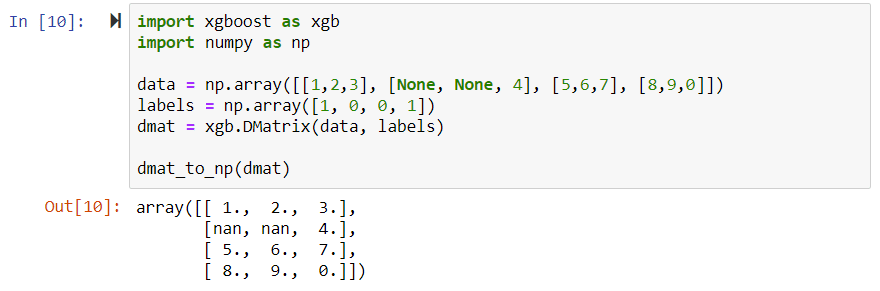### Step 5: Profiling & Reliability

It was great to find a solution but we could not yet rest on our laurels. It was crucial to make sure the conversion process time from DMatrix format to NumPy array would be similar to the opposite conversion direction.

We created a significantly larger matrix for out testing so we could detect time differences and used cProfile and snakeviz to measure our performance, The results were disappointing :

NumPy array to DMatrix (total time = 0.0533 seconds):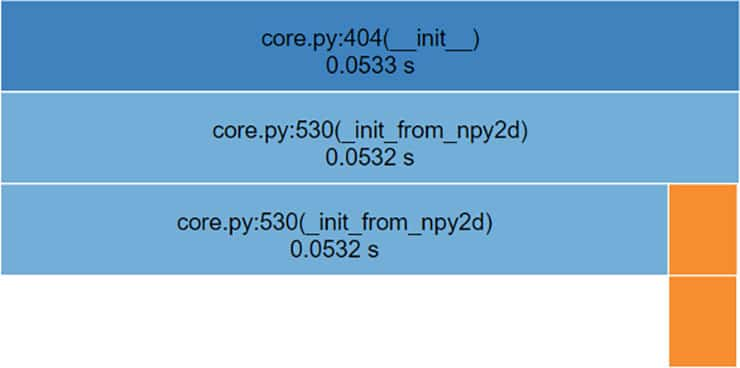Dmatrix to NumPy (total time = 78.3 seconds):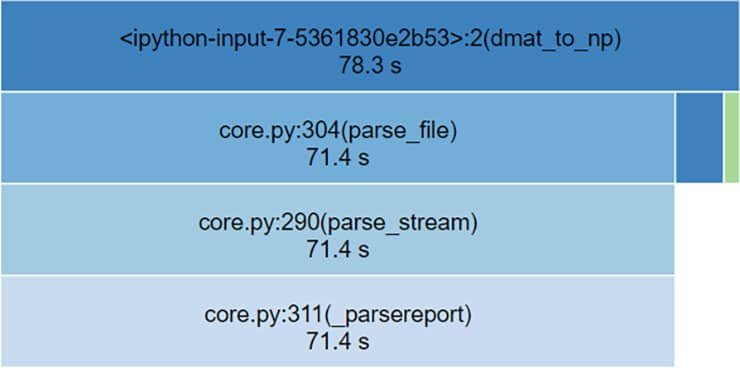We’ve tried to improve the construct library performance by following some recommendations from construct documentation, like compiled schema and lazy parsing. We even parsed the last data structure as a byte stream and accessed it by offsets.
These efforts weren’t fruitless but we were still too far from our goal of having similar conversion speed as in the opposite direction.

Something caught our attention in the construct library documentation. The benchmark comparison of Construct performance against the Kaiti library.

We checked the source code of the Kaiti Python library and realized that behind the scenes it was using the struct library! We decided to handle this task with our bare hands and manually parse the structure with direct reads from the file’s handle. Check our Github project to see the manual parsing solution

We’ve used an even larger matrix but with the manual parsing solution, the results were close enough, actually, not bad at all!

NumPy array to DMatrix (total time = 0.433 seconds):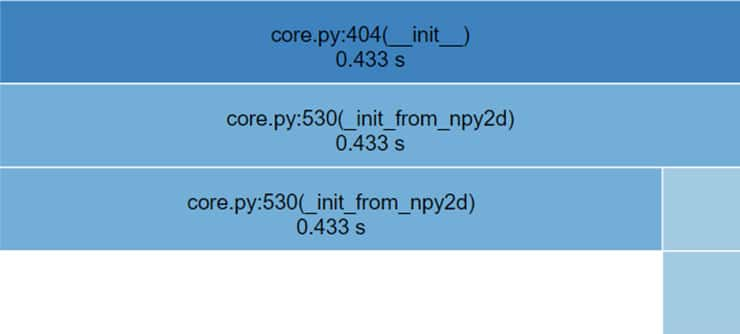Dmatrix to NumPy (total time = 0.764 seconds):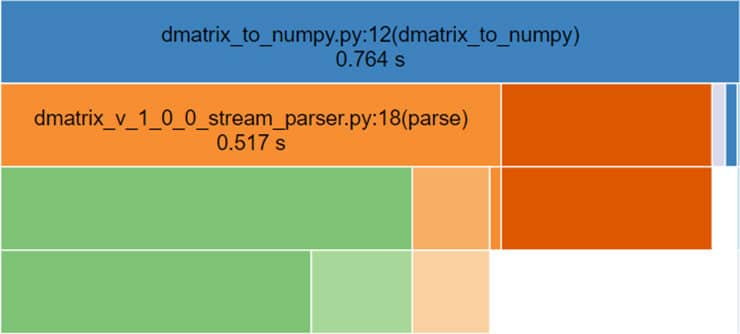### Don’t be numb! Go NumPy

The conversion task from Dmatrix to NumPy isn’t obvious but it’s certainly possible. The key to wrapping this process before sharing it with the world was reliability. It is crucial for our own machine learning productization system as well as to our clients’ standards.

We were strict about things like

• The differences between versions. We made a parsing algorithm for each version with even a slight difference.
• Byte ordering (endianness). Since we read and write the data from a file on the same platform, we made sure to read the file according to the platform’s endianness.
• Data Type Sizes – In cases where data type sizes like size_t or int are defined per platform, we used the struct library to know the data type size on the current platform
• Testing – we created a test matrix for all different XGBoost versions. For each version, NumPy matrices of different sizes, shapes, and contents (with certain edge cases) were converted to Dmatrix and back to check that the initial value is returned.

The project code is available in the following Github repository. Good luck!